# Pebble

The aquarium with internal dimensions of the bottom 40 cm × 35 cm and a height of 30 cm is filled with two-thirds of water. Calculate how many millimeters the water level in the aquarium rises by dipping a pebble-shaped sphere with a diameter of 18 cm.

Result

x =  21.812 mm

#### Solution:

$a = 40 \ cm \ \\ b = 35 \ cm \ \\ c = 30 \ cm \ \\ \ \\ c_{ 1 } = \dfrac{ 2 }{ 3 } \cdot \ c = \dfrac{ 2 }{ 3 } \cdot \ 30 = 20 \ cm \ \\ \ \\ D = 18 \ cm \ \\ r = D/2 = 18/2 = 9 \ cm \ \\ \ \\ V = \dfrac{ 4 }{ 3 } \cdot \ \pi \cdot \ r^3 = \dfrac{ 4 }{ 3 } \cdot \ 3.1416 \cdot \ 9^3 \doteq 3053.6281 \ cm^3 \ \\ V = abd \ \\ \ \\ d = V/(a \cdot \ b) = 3053.6281/(40 \cdot \ 35) \doteq 2.1812 \ cm \ \\ \ \\ d < c-c_{ 1 } \ \\ \ \\ x = d \rightarrow mm = d \cdot \ 10 \ mm = 21.8116289949 \ mm = 21.812 \ \text { mm }$

Leave us a comment of this math problem and its solution (i.e. if it is still somewhat unclear...):Math student
where did we get the small d?Dr Math
small d = height of water level rise. c is occupied yet, thus the next variable is d.#### Following knowledge from mathematics are needed to solve this word math problem:

Do you know the volume and unit volume, and want to convert volume units?

## Next similar math problems:

1. Cheops pyramid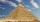The Pyramid of Cheops is a pyramid with a square base with a side of 233 m and a height of 146.6 m. It made from limestone with a density of 2.7 g/cm3. Calculate the amount of stone in tons. How many trains with 30 twenty tons wagons carry the stone?
2. Floating of wood - Archimedes lawWhat will be the volume of the floating part of a wooden (balsa) block with a density of 200 kg/m3 and a volume of 0.02 m3 that floats in alcohol? (alcohol density is 789 kg/m3)
3. Cuboid face diagonalsThe lengths of the cuboid edges are in the ratio 1: 2: 3. Will the lengths of its diagonals be the same ratio? The cuboid has dimensions of 5 cm, 10 cm, and 15 cm. Calculate the size of the wall diagonals of this cuboid.
4. Body diagonalCalculate the volume of a cuboid whose body diagonal u is equal to 6.1 cm. Rectangular base has dimensions of 3.2 cm and 2.4 cm
5. Parallels and one secant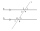There are two different parallel lines a, b and a line c that intersect the two parallel lines. Draw a circle that touches all lines at the same time.
6. FlowerbedWe enlarge the circular flower bed, so its radius increased by 3 m. The substrate consumption per enlarged flower bed was (at the same layer height as before magnification) nine times greater than before. Determine the original flowerbed radius.
7. Positional energyWhat velocity in km/h must a body weighing 60 kg have for its kinetic energy to be the same as its positional energy at the height 50 m?
8. Cylinder and its circumferenceIf the height of a cylinder is 4 times its circumference. What is the volume of the cylinder in terms of its circumference, c?
9. Surface of the cylinderCalculate the surface of the cylinder for which the shell area is Spl = 20 cm2 and the height v = 3.5 cm
10. The trapeziumThe trapezium is formed by cutting the top of the right-angled isosceles triangle. The base of the trapezium is 10 cm and the top is 5 cm. Find the area of trapezium.
11. Blueberries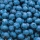5 children collect 4 liters of blueberries in 1.5 hours. a) How many minutes do 3 children take 2 liters of blueberries? b) How many liters of blueberries will be taken by 8 children in 3 hours?
12. AM of three numbersThe number 2010 can be written as the sum of 3 consecutive natural numbers. Determine the arithmetic mean of these numbers.
13. Propeller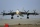The aircraft propeller rotates at an angular speed of 200 rad/s. A) What is the speed at the tip of the propeller if its distance from the axis of rotation is 1.5 m? B) What path does the aircraft travel during one revolution of the propeller at a speed
14. Reducing balance method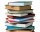A company buys an item having a useful life of 10 years for 1,000,000. If the company depreciates the item by the reducing balance method, a. Determine the depreciation for the first year. b. Estimate the depreciation for the second and third years. c..
15. Cut trees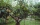There are 1,200 trees in the grove, of which 55 percent are deciduous, the remaining coniferous. Workers cut down 35 percent a) trees, b) deciduous trees, c) coniferous trees. How many trees now have this grove?
16. Uphill garden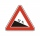I have a garden uphill, increasing from 0 to 4.5 m for a length of 25 m, how much is the climb in percent?
17. The escalator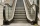I run up the escalator at a constant speed in the direction of the stairs and write down the number of steps A we climbed. Then we turn around and run it at the same constant speed in the opposite direction and write down the number of steps B that I climb